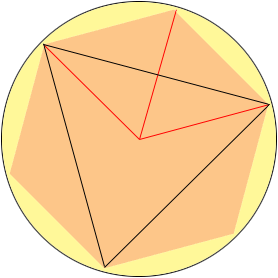SEARCH HOMEMath Central Quandaries & QueriesQuestion from Beth, a parent: A regular hexagon and an equilateral triangle are both inscribed in the same circle so that the hexagon and the triangle share three vertices. The radius of the circle is 10cm. What is the difference between the area of the hexagon and the area of the triangle?Hi Beth,

Draw lines from the center of the circle to the vertices of the hexagon. (I only drew three of them.) This cuts the hexagon into six congruent equilateral triangles. Two adjacent triangles form a rhombus whose area is cut in half by a side of the inscribed triangle.ChrisMath Central is supported by the University of Regina and The Pacific Institute for the Mathematical Sciences.